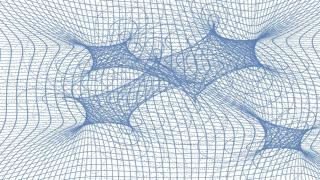# Microlensing Analysis for the Gravitational Lens Systems SDSS0924+0219, Q1355-2257, and SDSS1029+2623

Rojas, K.; Motta, V.; Mediavilla, E.; Jiménez-Vicente, J.; Falco, E.; Fian, C.
Bibliographical reference

The Astrophysical Journal

2
2020
Number of authors
6
IAC number of authors
2
Citations
12
Refereed citations
10
Description
We use spectroscopic observations of the gravitationally lensed systems SDSS0924+0219(BC), Q1355-2257(AB), and SDSS1029+2623(BC) to analyze microlensing and dust extinction in the observed components. We detect chromatic microlensing effects in the continuum and microlensing in the broad emission line profiles of the systems SDSS0924+0219(BC) and Q1355-2257(AB). Using magnification maps to simulate microlensing and modeling the emitting region as a Gaussian intensity profile with size rs ∝ λp, we obtain the probability density functions for a logarithmic size prior to ${\lambda }_{\mathrm{rest} \mbox{-} \mathrm{frame}}=3533\,\mathring{\rm A}$ . In the case of SDSS0924+0219, we obtain ${r}_{s}={4}_{-2}^{+3}\,\sqrt{M/{M}_{\odot }}$ lt-d (at 1σ), which is larger than the range of other estimates, and p = 0.8 ± 0.2 (at 1σ), which is smaller than predicted by the thin disk theory, but still in agreement with previous results. In the case of Q1355-2257 we obtain (at 1σ) ${r}_{s}={3.6}_{-1.6}^{+3.0}\,\sqrt{M/{M}_{\odot }}$ lt-d, which is also larger than the theoretical prediction, and p = 2.0 ± 0.7, which is in agreement with the theory within errors. SDSS1029+2326 spectra show evidence of extinction, probably produced by a galaxy in the vicinity of image C. Fitting an extinction curve to the data we estimate ∆E ̃ 0.2 in agreement with previous results. We found no evidence of microlensing for this system.
Related projectsRelativistic and Theoretical Astrophysics

Introduction Gravitational lenses are a powerful tool for Astrophysics and Cosmology. The goals of this project are: i) to obtain a robust determination of the Hubble constant from the time delay measured between the images of a lensed quasar; ii) to study the individual and statistical properties of dark matter condensations in lens galaxies from

Evencio